# Analog-to-Digital and Digital-to-Analog Converters

## Analog-to-Digital and Digital-to-Analog Converters

The world is analog, but signal processing nowadays is digital. The transition between the two domains is done using analog-to-digital (A/D) and digital-to-analog (D/A) converters.

Connecting digital circuitry to sensor devices is simple if the sensor devices are inherently digital themselves.

Switches, relays, and encoders are easily interfaced with gate circuits due to the on/off nature of their signals.

However, when analog devices are involved, interfacing becomes much more complex.

Hence, we need a way to electronically translate analog signals into digital (binary) quantities, and vice versa.

An analog-to-digital converter, or ADC, performs the former task while a digital-to-analog converter, or DAC, performs the latter.

An analog to digital converter (ADC, A/D, or A to D) is a device that converts a continuous physical quantity (usually voltage) to a digital number that represents the quantity’s amplitude.In block diagram form, it can be represented as such: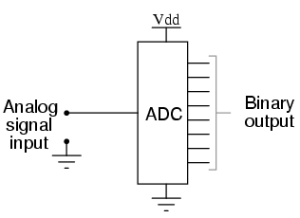Fig.1

A DAC, on the other hand, inputs a binary number and outputs an analog voltage or current signal.

In block diagram form, it looks like as below: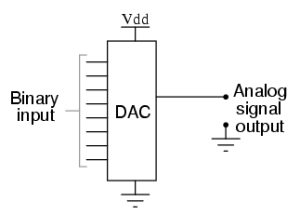Fig.2

Together, they are often used in digital systems to provide complete interface with analog sensors and output devices for control systems such as those used in automotive engine controls: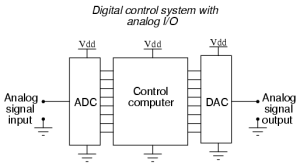Fig.3

It is much easier to convert a digital signal into an analog signal than it is to do the reverse. Therefore, we will begin with DAC circuitry and then move to ADC circuitry.

### Digital to Analog Converter using Binary-Weighted Resistors

A D/A converter using binary-weighted resistors is shown in the figure below.

In the circuit, the op-amp is connected in the inverting mode.The op-amp can also be connected in the non-inverting mode.

The circuit diagram represents a 4-digit converter. Thus, the number of binary inputs is four.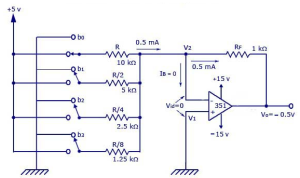Fig.4

We know that, a 4-bit converter will have 24 = 16 combinations of output. Thus, a corresponding 16 outputs of analog will also be present for the binary inputs.

#### Working

The circuit is basically working as a current to voltage converter.

#### When b0 is closed:

• It will be connected directly  to the +5V. Thus, voltage across R = 5V.
• So, current through R = 5V/10KΩ = 0.5mA.
• Current through feedback resistor, Rf = 0.5mA (Since, Input bias current, IB is negligible).
• Thus, output voltage = -(1KΩ)×(0.5mA) = -0.5V.

#### When b1 is closed, and b0 is open:

• R/2 will be connected to the positive supply of the +5V.
• So current through the resistor will become 5V/5KΩ = 1mA to flow through Rf.
• Thus, output voltage also doubles and Output voltage = -(1KΩ)×(1mA)= -1V.

#### When b0 and b1 are closed:

• Current through Rf = 1.5mA
• Hence, output voltage = -(1kohm)*(1.5mA) = -1.5V

Thus, according to the position (ON/OFF) of the switches (bo-b3), the corresponding “binary-weighted” currents will be obtained in the input resistor.

The current through Rf will be the sum of these currents.

This overall current is then converted to its proportional output voltage.

The output will be maximum if the switches (b0-b3) are closed.

#### Output voltage equation is given below: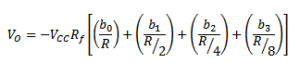The graph with the analog outputs versus possible combinations of inputs is shown below.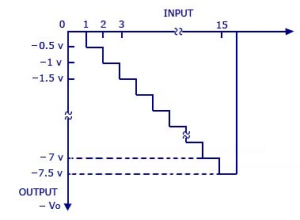Fig.5

The output is a negative going staircase waveform with 15 steps of -0.5V each.

In practice, due to the variations in the logic HIGH voltage levels, all the steps will not have the same size.

The value of the feedback resistor Rchanges the size of the steps. Thus, a desired size for a step can be obtained by connecting the appropriate feedback resistor.

The only condition to look out for is that the maximum output voltage should not exceed the saturation levels of the op-amp.

If the number of inputs (>4) or combinations (>16) is more, the binary-weighted resistors may not be readily available. This is why; R and 2R method is more preferred as it requires only two sets of precision resistance values.

### Digital to Analog Converter with R and 2R Resistors

A D/A converter with R and 2R resistors is shown in the figure below.

As in the binary-weighted resistors method, the binary inputs are applied by the switches (b0-b3), and the output is proportional to the binary inputs.

Binary inputs can be either in the HIGH (+5V) or LOW (0V) state. Let b3 be the most significant bit and thus is connected to the +5V and all the other switchs are connected to the ground.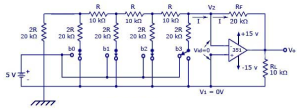Fig.6

Thus, according to Thevenin’s equivalent resistance, RTH,

RTH = [{[(2RII2R + R)} II2R] + R}II2R] + R = 2R = 20kOhms.

The resultant circuit is shown below.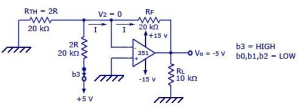Fig.7

Graph is given below.Fig.8

In the figure shown above, the negative input is at virtual ground, therefore the current through RTH=0.

Current through 2R connected to +5V = 5V/20KΩ = 0.25 mA

The current will be the same as that in Rf.

Vo = -(20KΩ)*(0.25mA) = -5V.

#### Output voltage equation is given below: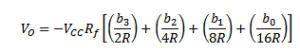### Flash Analog to Digital Converter

It is also known as parallel A/D converter and is formed of a series of comparators, each one comparing the input signal to a unique reference voltage.

The comparator outputs connect to the inputs of a priority encoder circuit, which then produces a binary output.

The following example shows a 3-bit flash ADC circuit: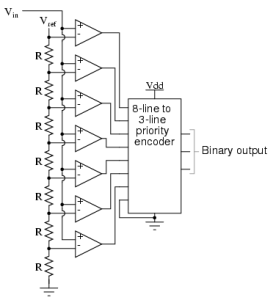Fig.9

Vref is a stable reference voltage provided by a precision voltage regulator as part of the converter circuit, not shown in diagram.

As the analog input voltage exceeds the reference voltage at each comparator, the comparator outputs will sequentially saturate to a high state.

The priority encoder generates a binary number based on the highest-order active input, ignoring all other active inputs.

When operated, the flash ADC produces an output that looks something like this: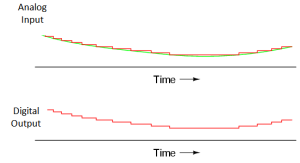Fig.10

For this particular application, a regular priority encoder with all its inherent complexity isn’t necessary.

It may be realized through a set of Exclusive-OR gates, allowing the use of a simpler, non-priority encoder as shown below in Fig.12.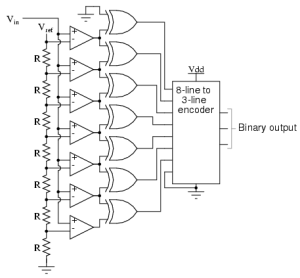Fig.11

The flash converter is not only the simplest in terms of operational theory, but it is the most efficient  in terms of speed, being limited only in comparator and gate propagation delays.

Unfortunately, it is the most component-intensive for any given number of output bits.

The three-bit flash ADC requires seven comparators. A four-bit version would require 15 comparators. With each additional output bit, the number of required comparators doubles.

Considering that eight bits is generally considered the minimum necessary for any practical ADC (255 comparators needed), the flash methodology quickly shows its weakness.

### Digital Ramp Analog to Digital Converter

It is also known as the stairstep-ramp, or simply counter A/D converter.

The basic idea of this ADC, is to connect the output of a free-running binary counter to the input of a DAC, then compare the analog output of the DAC with the analog input signal to be digitized and use the comparator’s output to tell the counter when to stop counting and reset.

The following schematic shows the basic idea: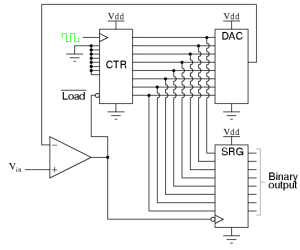Fig.12

#### Operation

As the counter counts up with each clock pulse, the DAC outputs a slightly higher (more positive) voltage.

This voltage is compared against the input voltage by the comparator.

If the input voltage is greater than the DAC output, the comparator’s output will be high and the counter will continue counting normally.

Eventually, though, the DAC output will exceed the input voltage, causing the comparator’s output to go low. This will cause two things to happen:

• first, the high-to-low transition of the comparator’s output will cause the shift register to “load” whatever binary count is being output by the counter, thus updating the ADC circuit’s output.
• secondly, the counter will receive a low signal on the active-low LOAD input, causing it to reset to 00000000 on the next clock pulse.

The effect of this circuit is to produce a DAC output that ramps up to whatever level the analog input signal is at, output the binary number corresponding to that level, and start over again.

The input and output waveform of a Digital Ramp ADC is shown below: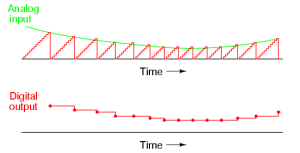Fig.13

Note that the time between the digital output values changes depending on how high the input voltage is.

For low signal levels, the digital outputs are rather close-spaced. For higher signal levels, they are spaced further apart in time: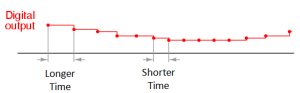Fig.14

For many ADC applications, this variation in sample time would not be acceptable.

This circuit need to count all the way from 0 at the beginning of each count cycle makes for relatively slow sampling of the analog signal, places the digital-ramp ADC at a disadvantage.

### Successive Approximation Type Analog to Digital Converter

The only change in this design is a very special counter circuit known as a successive-approximation register.

Instead of counting up in binary sequence, this register counts by trying all values of bits starting with the most-significant bit and finishing at the least-significant bit.

Throughout the count process, the register monitors the comparator’s output to see if the binary count is less than or greater than the analog signal input, adjusting the bit values accordingly.

The way the register counts is identical to the “trial-and-fit” method of decimal-to-binary conversion, whereby different values of bits are tried from MSB to LSB to get a binary number that equals the original decimal number.

The advantage to this counting strategy is much faster results: the DAC output converges on the analog signal input in much larger steps than with the 0-to-full count sequence of a regular counter.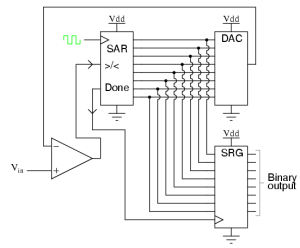Fig.15

It should be noted that the SAR is generally capable of outputting the binary number in serial (one bit at a time) format, thus eliminating the need for a shift register.

Plotted over time, the operation of a successive-approximation ADC is as shown below: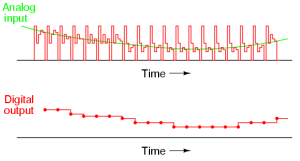Fig.16

It can be noted that  the updates for this ADC occur at regular intervals, unlike the digital ramp ADC circuit.